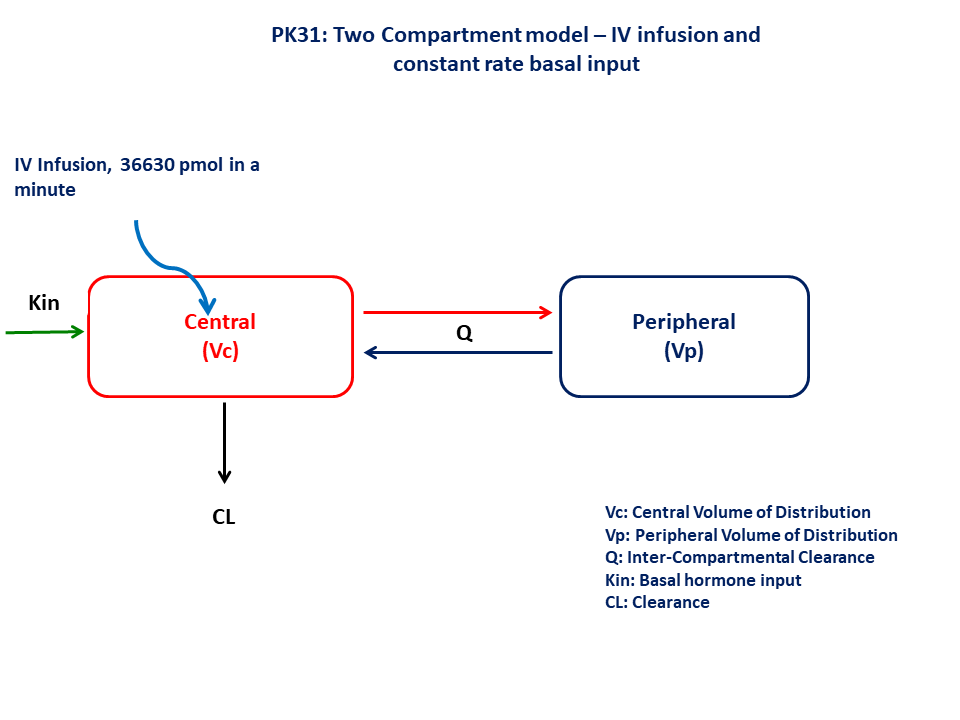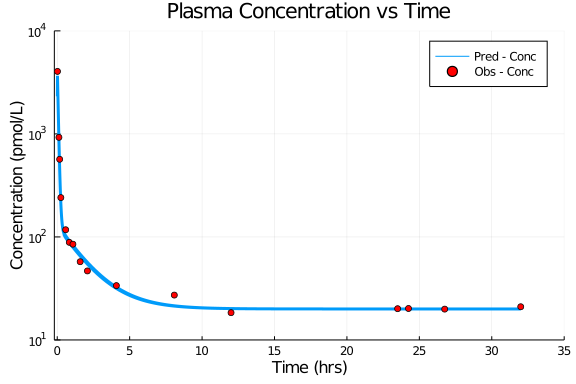# Exercise - PK31 Turnover II - Intravenous dosing of hormone

### Background

• Structural model - Two compartment with additional input for basal hormone synthesis in the central compartment

• Route of administration - IV infusion (1 minute)

• Dosage Regimen - 36,630 pmol

• Number of Subjects - 1### Learning Outcome

In this model, you will learn how to build a two compartment with additional input for basal hormone level. This model will help to simulate the plasma concentration profile after IV administration considering basal hormone input.

### Objectives

• To analyze the intavenous datasets with parallel turnover

• To write multi-compartment model in terms of dfferential equations

### Libraries

call the "necessary" libraries to get start.

using Pumas
using Plots
using CSV
using StatsPlots
using Random


### Model

In this one compartment model PK31, we administer IV infusion dose to central compartment.

pk_31           = @model begin
@param begin
tvkin       ∈ RealDomain(lower=0)
tvvc        ∈ RealDomain(lower=0)
tvcl        ∈ RealDomain(lower=0)
tvq         ∈ RealDomain(lower=0)
tvvp        ∈ RealDomain(lower=0)
Ω           ∈ PDiagDomain(5)
σ²_prop     ∈ RealDomain(lower=0)

end

@random begin
η           ~ MvNormal(Ω)
end

@pre begin
Kin         = tvkin * exp(η)
Vc          = tvvc* exp(η)
Cl          = tvcl * exp(η)
Q           = tvq  * exp(η)
Vp          = tvvp * exp(η)
end

@dynamics begin
Central'    =  Kin  - (Cl/Vc)*Central - (Q/Vc)*Central + (Q/Vp)*Peripheral
Peripheral' = (Q/Vc)*Central - (Q/Vp)*Peripheral
end

@derived begin
cp          = @. Central/Vc
dv          ~ @. Normal(cp, sqrt(cp^2*σ²_prop))
end
end

PumasModel
Parameters: tvkin, tvvc, tvcl, tvq, tvvp, Ω, σ²_prop
Random effects: η
Covariates:
Dynamical variables: Central, Peripheral
Derived: cp, dv
Observed: cp, dv


### Parameters

The parameters are as given below. tv represents the typical value for parameters.

• Kin - Basal hormonal input (pmol/hr)

• Vc - Central Volume of Distribution (L)

• Cl - Clearance (L/hr)

• Q - Intercompartmental Clearance (L/hr)

• Vp - Peripheral Volume of Distribution (L)

• Ω - Between Subject Variability,

• σ - Residual error

param = ( tvkin   = 1531.87,
tvvc    = 8.8455,
tvcl    = 76.5987,
tvq     = 56.8775,
tvvp    = 58.8033,
Ω       = Diagonal([0.0,0.0,0.0,0.0,0.0]),
σ²_prop = 0.015)

(tvkin = 1531.87, tvvc = 8.8455, tvcl = 76.5987, tvq = 56.8775, tvvp = 58.8
033, Ω = [0.0 0.0 … 0.0 0.0; 0.0 0.0 … 0.0 0.0; … ; 0.0 0.0 … 0.0 0.0; 0.0
0.0 … 0.0 0.0], σ²_prop = 0.015)


### Dosage Regimen

A single dose of 36630 pmol is given as an IV Infusion

ev1  = DosageRegimen(36630, time=0, cmt=1, duration=0.0166)
sub1 = Subject(id=1, events=ev1)

Subject
ID: 1
Events: 2


### Simulation

Simulate the plasma concentration profile

Random.seed!(123)
sim_sub1 = simobs(pk_31, sub1, param , obstimes=0.01:0.0001:32)


### Dataframe & Plot

Convert the simulation to a dataframe and use it for plotting.

df1    = DataFrame(sim_sub1)
df1_dv = filter(x -> x.time in [0.0167,0.1167,0.167,0.25,0.583,0.833,1.083,1.583,2.083,4.083,8.083,12,23.5,24.25,26.75,32], df1)

@df df1 plot(:time, :cp, yaxis=:log,
label="Pred - Conc",  xlabel="Time (hrs)", ylabel="Concentration (pmol/L)",
title="Plasma Concentration vs Time", linewidth=3,
xlims=(-0.2,35), xticks=[0,5,10,15,20,25,30,35], yticks=[10,100,1000,10000], ylims=(10,10000))
@df df1_dv scatter!(:time, :dv, label="Obs - Conc", color=[:red])